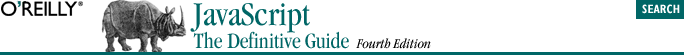home | O'Reilly's CD bookshelfs | FreeBSD | Linux | Cisco | Cisco Exam13.9. The Location Object

Example 13-5. Extracting arguments from a URL

/*
* This function parses comma-separated name=value argument pairs from
* the query string of the URL. It stores the name=value pairs in
* properties of an object and returns that object.
*/
function getArgs( ) {
var args = new Object( );
var query = location.search.substring(1);     // Get query string
var pairs = query.split(",");                 // Break at comma
for(var i = 0; i < pairs.length; i++) {
var pos = pairs[i].indexOf('=');          // Look for "name=value"
var argname = pairs[i].substring(0,pos);  // Extract the name
var value = pairs[i].substring(pos+1);    // Extract the value
args[argname] = unescape(value);          // Store as a property
// In JavaScript 1.5, use decodeURIComponent( ) instead of escape( )
}
return args;                                  // Return the object
}

/*
* We could have used getArgs( ) in the previous bouncing window example
* to parse optional animation parameters from the URL
*/
var args = getArgs( );                   // Get arguments
if (args.x) x = parseInt(args.x);         // If arguments are defined...
if (args.y) y = parseInt(args.y);         // override default values
if (args.w) w = parseInt(args.w);
if (args.h) h = parseInt(args.h);
if (args.dx) dx = parseInt(args.dx);
if (args.dy) dy = parseInt(args.dy);
if (args.interval) interval = parseInt(args.interval);
// If the user is using an old browser that can't display DHTML content,
// redirect to a page that contains only static HTML
if (parseInt(navigator.appVersion) < 4)
location = "staticpage.html";